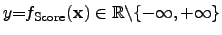## 4.4.2 Selection of Optimization Strategies and Score Functions

An appropriate selection of the score function decreases the iteration necessary for the optimization and often increases the quality of the optimization result. However, the construction of the score function with the selections of the quality criteria is even more complex than the simulation itself and thus often compared to an art because visually obvious selection criteria have to be described by mathematical equations to select the relevant data out of the simulation results available. The optimal optimization strategy can be described as that one which yields the global optimum within the shortest period of time. However, this assumes that the problem class for the optimization is known, that all constraints can be well formulated, and that the response surface is rather smooth. In realistic applications, these assumptions are often not valid. Most problem descriptions operate at a certain modeling level, where the model is also constructed out of a set of assumptions. In addition, many models check if the operating bias point is still in the region for which the assumption has been set. If not, the model behavior can be switched and the behavior is in general no longer smooth. However, there are certain decision criteria for the selection of optimization strategies according to . If sufficient information about the simulation tools is available, the design engineer may estimate the shape of the response surface with respect to the input parameters. With that information it is possible to select one optimization strategy out of the pool of the available optimizers.

Most optimizers provide at least one termination criterion which is the maximum number of parameter evaluations. However, if the quality cannot be appropriately measured or determined, the best optimization algorithm cannot converge to a good result if the score function produces too much noise, checks or extracts the wrong quantities, or even diverges. The experience over the last decades has shown, that the most commonly used score functions are the EUCLIDian norm (2-norm), the minimum norm, and the maximum norm. All of those score functions converge if the quantity consists of finite real numbersand if there exists at least one local minimum. However, the choice of the score function determines the speed of convergence.

Stefan Holzer 2007-11-19